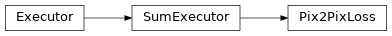# Pix2PixLoss¶

Inheritance Diagramclass ashpy.losses.gan.Pix2PixLoss(l1_loss_weight=100.0, adversarial_loss_weight=1.0, feature_matching_weight=10.0, adversarial_loss_type=<AdversarialLossType.GAN: 0>, use_feature_matching_loss=False)[source]

Pix2Pix Loss.

Used by Pix2Pix  and Pix2PixHD 

1

Image-to-Image Translation with Conditional Adversarial Networks https://arxiv.org/abs/1611.07004

2

High-Resolution Image Synthesis and Semantic Manipulation with Conditional GANs https://arxiv.org/abs/1711.11585

Methods

 __init__([l1_loss_weight, …]) Initialize the loss.

Attributes

 executors Return the List of Executors. fn Return the Keras loss function to execute. global_batch_size Global batch size comprises the batch size for each cpu. weight Return the loss weight.
__init__(l1_loss_weight=100.0, adversarial_loss_weight=1.0, feature_matching_weight=10.0, adversarial_loss_type=<AdversarialLossType.GAN: 0>, use_feature_matching_loss=False)[source]

Initialize the loss.

Parameters
• l1_loss_weight (ashpy.ashtypes.TWeight) – Weight of L1 loss.

• adversarial_loss_weight (ashpy.ashtypes.TWeight) – Weight of adversarial loss.

• feature_matching_weight (ashpy.ashtypes.TWeight) – Weight of the feature matching loss.

• adversarial_loss_type (ashpy.losses.gan.AdversarialLossType) – Adversarial loss type (ashpy.losses.gan.AdversarialLossType.GAN or ashpy.losses.gan.AdversarialLossType.LSGAN).

• use_feature_matching_loss (bool) – if True use also uses ashpy.losses.gan.FeatureMatchingLoss.

Return type

None